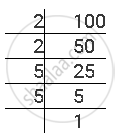# The Following Numbers Are Not Perfect Cubes? 100 - Mathematics

#### Question

The following numbers are not perfect cubes?

100

#### Solution

The prime factorisation of 100 is as follows.100 = 2 × 2 × 5 × 5

Here, each prime factor is not appearing as many times as a perfect multiple of 3. Two 2s and two 5s are remaining after grouping the triplets. Therefore, 100 is not a perfect cube

Is there an error in this question or solution?

#### APPEARS IN

NCERT Solution for Mathematics Textbook for Class 8 (2018 (Latest))
Chapter 7: Cubes and Cube Roots
Exercise 7.1 | Q 1.4 | Page 114

#### Video TutorialsVIEW ALL 

The Following Numbers Are Not Perfect Cubes? 100 Concept: Some Interesting Patterns of Cube Numbers.